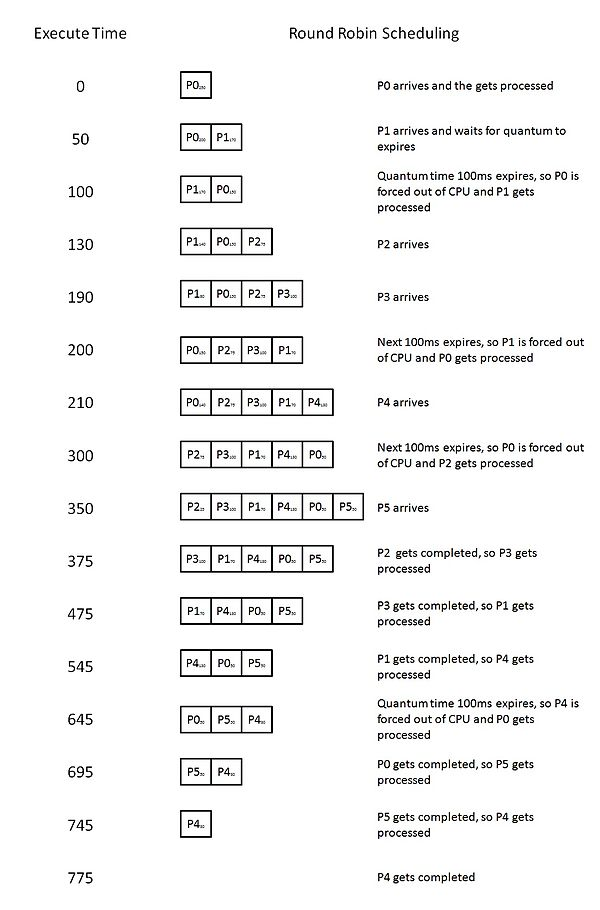×

Search anything:

# Round Robin Scheduling Algorithm

#### Software Engineering AlgorithmsReading time: 25 minutes | Coding time: 10 minutes

Round Robin Scheduling Algorithm is one of the simplest scheduling algorithm used in various operating systems for process scheduling and networks. The key idea is to allocate CPU to all processes in the same order for the same amount of time.

It is also a preemptive scheduling algorithm famous for CPU Scheduling and used in various Operating Systems. It is better than other approaches like Shortest Job First algorithms considering that there is a guarantee that all processes will be completed at the cost of overall performance but it is better than brute force approach.

In fact, Round Robin scheduling algorithm is one of the first algorithms to provide a real time computing experience.

## Algorithm Explanation

The aim of this algorithm is to determine or schedule the order of execution of the processes. Now first each process is given with a fixed amount of time period known as quanta , this time quantum remains fixed throughout the process.

Each process can only be executed for one quanta at a time if the process manages to complete the execution within this time then it is terminated else it is preempted and has to wait for it's turn in a circular order to execute again, here context switching is used to save states of the preempted processes.

## Advantages of Round Robin Algorithm

• Because of the fixed quantum time each and every process has the same priority.
• No stagnation or starvation of processes occurs, no process gets left behind.
• There will be a improvement in average response time of the processes.
• CPU is best utilized, no need of any extra resources.
• Threads having same priority are scheduled perfectly with Round Robin as CPU is equally shared between all processes.

## Disadvantages of Round Robin Algorithm

• Higher average waiting time because every process has to wait until it's turn.
• The length of quantum time has a large effect on the waiting time, too less will cause more time waste in switching through the processes than executing them effectively.
• Low Throughput, means decreased rate of output or completion as a process is not completely executed at a time.
• Lack of priority might be a problem in cases with processes which require immediate attention.

## Example of Round Robin Algorithm

Consider the following example with five processes each one with varied execution time and a quantum time of 100 ms

Process Name Arrival Time Execution Time
P0 0 250
P1 50 170
P2 130 75
P3 190 100
P4 210 130
P5 350 50
1. First, each and every process gets a quantum time of 100 ms.
2. Note that the processes P2 and P5 which have an execution time less than the quantum time hence once their execution is complete they are terminated and the next process takes over.
3. Other process which have execution time more than 100 ms will take multiple turns to complete their execution process.

Now the scheduling will be as follows :### Program of Round Robin Algorithm in C++

Following is the C++ implementation of Round Robin scheduling algorihm (go through it carefully):

``````// Part of OpenGenus IQ
#include<stdio.h>
#include<conio.h>
#include<process.h>
#include<string.h>
void main()
{
// p -> process
char p;
// et -> execution time for current turn
// wt -> waiting time
// pt -> processing time (taken as input)
int et, wt, timer=3, count, pt, rt;
int i, j, totwt=0, t, n=5, found=0, m;
float avgwt;
clrscr();

// Read the process name and processing time
for(i=0;i<n;i++)
{
printf("enter the process name : ");
scanf("%s",&p[i]);
printf("enter the processing time : ");
scanf("%d",&pt[i]);
}

m=n;
wt = 0;
i=0;

// Scheduling starts
do
{
if(pt[i]>timer)
{
rt=pt[i]-timer;
strcpy(p[n],p[i]);
pt[n]=rt;
et[i]=timer;
n++;
}
else
{
et[i]=pt[i];
}
i++;
wt[i]=wt[i-1]+et[i-1];
} while(i<n);

count=0;
for(i=0;i<m;i++)
{
for(j=i+1;j<=n;j++)
{
if(strcmp(p[i],p[j])==0)
{
count++;
found=j;
}
}
if(found!=0)
{
wt[i]=wt[found]-(count*timer);
count=0;
found=0;
}
}
for(i=0;i<m;i++)
{
totwt+=wt[i];
}
avgwt=(float)totwt/m;

// Display the Result
for(i=0;i<m;i++)
{
printf("\nProcess Name\tProcessing Time\tWaiting Time");
printf("\n%s\t%d\t%d",p[i],pt[i],wt[i]);
}
printf("\nTotal waiting time %d\n",totwt);
printf("Average waiting time %f",avgwt);
}
``````

Output

INPUT :

Enter the process name : A
Enter the processing time : 4
Enter the process name : B
Enter the processing time : 3
Enter the process name : C
Enter the processing time : 2
Enter the process name : D
Enter the processing time : 5
Enter the process name : E
Enter the processing time : 1

OUTPUT :

Process Name Processing Time Waiting Time
A 4 9
B 3 3
C 2 6
D 5 10
E 1 11

Total waiting time : 39
Average waiting time : 7.8000

## Thoughts

Round Robin Scheduling algorithm may seem to be a simple algorithm but it is mathematically proven to be a decent approach. Compare this with a greedy algorithm like Shortest Job First Scheduling.

In such greedy algorithms, there may be processes which may never complete in the cost of immediate better performance. In case of Round Robin Scheduling, though the overall performance may be worst but there is a guarantee that everything will be completed.

This is an important approach as it shows how simple algorithms can be designed. Think of this algorithmic approach in other domains and you will get to understand deeper Computer Science concepts.

With this, you have the complete knowledge of Round Robin Scheduling algorithm. Enjoy.#### Abhiram Reddy Duggempudi

Abhiram Reddy Duggempudi has been an Algorithm and Data Structure Developer, Intern at OpenGenus and is a Student at Sathyabama Institute of Science and Technology.

Round Robin Scheduling Algorithm